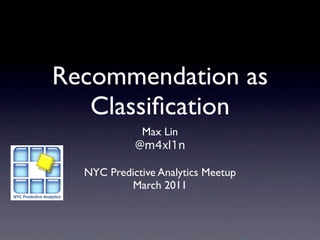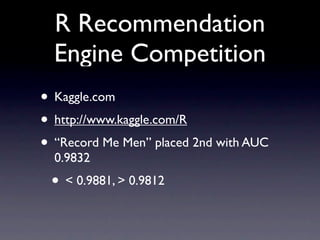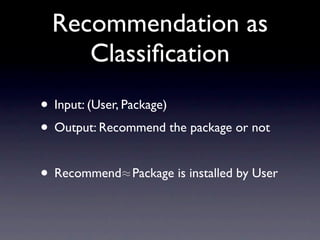Successfully reported this slideshow.

# Recommendation as Classification

6

Share×
1 of 16
1 of 16

# Recommendation as Classification

6

Share

#### Description

Max Lin presented at the NYC Predictive Analytics meetup on his submission to the R recommendation engine competition at Kaggle.

#### Transcript

1. 1. Recommendation as Classiﬁcation Max Lin @m4xl1n NYC Predictive Analytics Meetup March 2011
2. 2. R Recommendation Engine Competition • Kaggle.com • http://www.kaggle.com/R • “Record Me Men” placed 2nd with AUC 0.9832 • < 0.9881, > 0.9812
3. 3. Recommendation as Classiﬁcation • Input: (User, Package) • Output: Recommend the package or not • Recommend≈ Package is installed by User
4. 4. Classiﬁcation • Features • Classiﬁer training algorithms • Training: Minimize loss + regularizers J(θ) = L(yi , f (ui , pi ; θ)) + λR(θ) • Stochastic gradient descent i • Choose parameters by cross validation
5. 5. Classiﬁcation Models • Model 1: Baseline • Model 2: Latent factor models • Model 3: Package LDA topic • Model 4: Package task view • Ensemble Learning
6. 6. M1: Baseline • Provided by the contest organizer • Strong baseline: AUC of ~0.94 • 7 package features + User factors • Logistic Regression
7. 7. M2: Factor Models • Features: user factors, package factors, latent user and package factors • Classiﬁer: f (u, p) = µ + µu + µp + T βu βp • Minimize exponential loss + L2 regularizers
8. 8. Model Expressiveness
9. 9. M3: Package LDA topic • Features: user factors, package factors, package LDA topics • Classiﬁer: Similar to M2 f (u, v) = µ + µu + µp + tu
10. 10. M4: Package task view • Features: user factors, package factors, package task views • e.g., high-performance computing • Classiﬁer: Similar to M3 f (u, v) = µ + µu + µp + tu
11. 11. Ensemble Learning • Combine predictions from individual models • Logistic Regression
12. 12. Code & More • Github https://github.com/m4xl1n • Python + R • Blog post: http://bit.ly/hWmQyM
13. 13. Lessons • Features, Features, Features • User factors, package factors • Data cleaning • Domain knowledge

• \n
• \n
• \n
• \n
• \n
• \n
• \n
• \n
• \n
• \n
• \n
• \n
• \n
• \n
• \n
• \n
• #### Description

Max Lin presented at the NYC Predictive Analytics meetup on his submission to the R recommendation engine competition at Kaggle.

#### Transcript

1. 1. Recommendation as Classiﬁcation Max Lin @m4xl1n NYC Predictive Analytics Meetup March 2011
2. 2. R Recommendation Engine Competition • Kaggle.com • http://www.kaggle.com/R • “Record Me Men” placed 2nd with AUC 0.9832 • < 0.9881, > 0.9812
3. 3. Recommendation as Classiﬁcation • Input: (User, Package) • Output: Recommend the package or not • Recommend≈ Package is installed by User
4. 4. Classiﬁcation • Features • Classiﬁer training algorithms • Training: Minimize loss + regularizers J(θ) = L(yi , f (ui , pi ; θ)) + λR(θ) • Stochastic gradient descent i • Choose parameters by cross validation
5. 5. Classiﬁcation Models • Model 1: Baseline • Model 2: Latent factor models • Model 3: Package LDA topic • Model 4: Package task view • Ensemble Learning
6. 6. M1: Baseline • Provided by the contest organizer • Strong baseline: AUC of ~0.94 • 7 package features + User factors • Logistic Regression
7. 7. M2: Factor Models • Features: user factors, package factors, latent user and package factors • Classiﬁer: f (u, p) = µ + µu + µp + T βu βp • Minimize exponential loss + L2 regularizers
8. 8. Model Expressiveness
9. 9. M3: Package LDA topic • Features: user factors, package factors, package LDA topics • Classiﬁer: Similar to M2 f (u, v) = µ + µu + µp + tu
10. 10. M4: Package task view • Features: user factors, package factors, package task views • e.g., high-performance computing • Classiﬁer: Similar to M3 f (u, v) = µ + µu + µp + tu
11. 11. Ensemble Learning • Combine predictions from individual models • Logistic Regression
12. 12. Code & More • Github https://github.com/m4xl1n • Python + R • Blog post: http://bit.ly/hWmQyM
13. 13. Lessons • Features, Features, Features • User factors, package factors • Data cleaning • Domain knowledge

• \n
• \n
• \n
• \n
• \n
• \n
• \n
• \n
• \n
• \n
• \n
• \n
• \n
• \n
• \n
• \n
• ## More Related Content

### Related Books

Free with a 30 day trial from Scribd

See all

### Related Audiobooks

Free with a 30 day trial from Scribd

See all